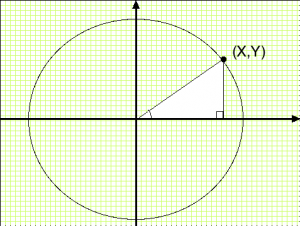•• # Are Diameter and Radius the Same

The answer to the question “are diameter and radius the same” is they are not. Diameter refers to the width of a circle. Radius is the closest distance by way of a straight line between a circle’s perimeter and its center point. A circle’s radius is half its diameter, so it is half the circle.

### How to Calculate Circle Radius and DiameterTo calculate this, you need to know a circle’s circumference. Circumference is the circle’s total distance around. It the same as diameter but multiplied by 3.14 pi. First, get the circle’s circumference.

If it is 12.56, divide that number by pi 3.14. The answer is 4. 4 is the circle diameter. To get the radius, divide the diameter number by 2. In this case, diameter 4 is divided by 2 which mean the radius is 2.

### Calculating Oval Radius and Diameter

Ovals seem like semi-circles, but the calculations are different. You need to figure out the semi minor and semi major axis. The precise steps are outlined below. Followed correctly you can get the results.

### The Semi Minor Axis

Calculate the distance from the oval’s perimeter to a focus point. Mark this A. In this example A=5 cm. Get the distance between the same perimeter point and another focus point. This is B and equals 3 cm. Add A and B and square the result. In this example that would be 5 cm + 3 cm equals 8 cm. 8 squared is 64 cm.

Get the distance between two focus points and get F. Square it. In this case that would be 25 cm with F=5. Subtract 25 cm from 64 cm. The result is 39. 39 square is 6.245 which is its shortest diameter. Get the radius by dividing in half the semi minor axis. 6.245 cm divided by 2 equals 3.122 cm.

### The Semi Major Axis

Perform the same process to get A and B. Assume the results are 5 and 3 cm. Add the two and you get the semi major axis. Half 8 by 2 to get the radius. In this case it is 4 cm. As you can see, the calculations are not the same. Although it seems complicated, the process is really quite simple.

Again, to answer the question “are diameter and radius the same”, the answer is they are not the same. Diameter is the circle distance through its middle. Radius is half of it.